# GSEB Solutions Class 6 Maths Chapter 3 Playing With Numbers Ex 3.7

Gujarat Board GSEB Textbook Solutions Class 6 Maths Chapter 3 Playing With Numbers Ex 3.7 Textbook Questions and Answers.

## Gujarat Board Textbook Solutions Class 6 Maths Chapter 3 Playing With Numbers Ex 3.7

Question 1.
Renu purchases two bags of fertiliser of weights 75 kg and 69 kg. Find the maximum value of weight which can measure the weight of the fertiliser exact number of times.
Solution:
The maximum value of weight which can measure the given weight exact number of time is their HCF.
Now, Factors of 75 are: 1, 3, 5, 15, 25 and 75 Factors of 69 are: 1, 3, 23 and 69
Common factors are: 1 and 3
HCF of 75 and 69 = 3
Thus, the required maximum value of weight = 3 kg.

Question 2.
Three boys step off together from the same spot. Their steps measure 63 cm, 70 cm and 77 cm respectively What is the minimum distance each should cover so that all can cover the distance in complete steps?
Solution:
The minimum distance each boy should walk must be the least common multiple of the measure of their steps.
To find LCM of 63, 70 and 77, we have: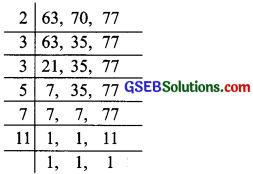LCM of 63, 10 and 17
= 2 x 3 x 3 x 5 x 7 x 11 = 6,930
The required minimum distance 6,930 cmQuestion 3.
The length, breadth and height of a room are 825 cm, 675 cm and 450 cm respectively. Find the longest tape which can measure the three dimensions of the room exactly.
Solution:
The longest tape will be the H.C.F of 825, 675 and 450.
We have: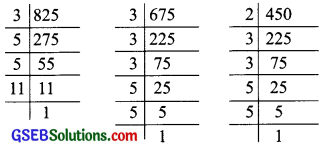825 = 3 x 5 x 5 x 11
675 = 3 x 3 x 3 x 5 x 5
450 = 2 x 3 x 3 x 5 x 5
HCF of 825, 675 and 450 = 3 x 5 x 5 = 75
Thus, the required length of the longest tape = 75cm.Question 4.
Determine the smallest 3-digit number which is exactly divisible by 6, 8 and 12.
Solution:
The LCM of 6, 8 and 12 is exactly divisible by them.
We have: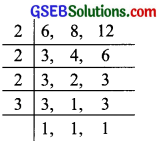LCM of 6,8 and 12 = 2 x 2 x 2 x 3 = 24
All other multiples of 24 will also be divisible by
6, 8 and 12. But we need the smallest multiple of 24 having 3-digits.
Since, the 3-digit smallest number = 100i.e. the multiple of 24 just above 100
= (100 – 4) + 24 or 120,
Hence, the required number 120.Question 5.
Determine the greatest 3-digit number exactly divisible by 8, 10 and 12.
Solution:
To find LCM of 8, 10 and 12, we have: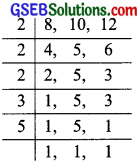LCM = 2 x 2 x 2 x 3 x 5 = 120
Since, all other multiples of 120 will also be divisible by 8, 10 and 12. But we need the greatest 3-digit number which is a multiple of 120.
We have:( 999 is the greatest 3-digit number.)
999 – 39, i.e. 960 is a multiple of 120.
Thus, the required number – 960Question 6.
The traffic lights at three different road crossings change after every 48 seconds, 72 seconds and 108 seconds respectively. If they change simultaneously at 7 a.m., at what lime will they change simultaneously again?
Solution:
The required time will be the LCM of 48, 72 and 108.
To find their LCM.
we have: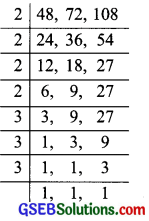LCM = 2 x 2 x 2 x 2 x 3 x 3 x 3 = 432
The required time is 432 seconds, i.e.
7 minutes and 12 seconds after 7 a.m.
[432 seconds = 7 minutes 12 seconds]
Thus, the required time = 7 minutes 12 seconds past 7 am.Question 7.
Three tankers contain 403 litres, 434 litres and 465 litres of diesel respectively. Find the maximum capacity of a container that can measure the diesel of the three containers exact number of times.
Solution:
The maximum capacity of the required measure will be equal to the HCF of 403, 434 and 465 litres.Factors of 403 are 1, 13, 31 and 403.
Factors of 434 are 1, 2, 7, 31 and 434.
Factors of 465 are 1, 3, 5, 31 and 465.
Their common factors are: 1 and 31
i.e. HCF = 31
Thus, the maximum capacity of the required container = 31 litres.Question 8.
Find the least number which when divided by 6, 15 and 18 leave remainder 5 in each case.
Solution:
We have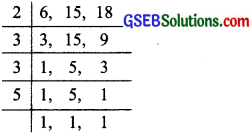LCM of 6, 15 and 18 = 2 x 3 x 3 x 5 = 90
Since, 90 is the least number exactly divisible
by 6, 15 and 18. To get a remainder 5, the least
number will be 90 + 5, i.e. 95. Thus, the required number = 95.Question 9.
Find the smallest 4-digit number which is divisible by 18, 24 and 32.
Solution:
We haveLCM = 2 x 2 x 2 x 2 x 2 x 3 x 3 = 288
Since, 288 is the smallest number which is
divisible by 18, 24 and 32. But it is not a 4-digit number,
Since, the smallest 4-digit number = 1000
Now,The multiple of 288 which is just above 1000 is 1000 – 136 + 288, i.e. 1152.
Thus, the required number = 1152.Question 10.
Find the LCM of the following numbers:
(a) 9 and 4
(b) 12 and 5
(c) 6 and 5
(d) 15 and 4
Observe a common property in the obtained LCMS. Is LCM the product of two numbers in each case?
Solution:
(a) 9 and 4
We haveLCM of 9 and 4 = 2 x 2 x 3 x 3 = 36
Since, product of 9 and 4 = 9 x 4 = 36,
LCM of 4 and 9 = product of 9 and 4

(b) 12 and 5
We have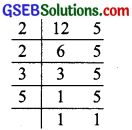LCM of 12 and 5 = 2 x 2 x 3 x 5 = 60
Since, the product of 12 and 5 = 60,
LCM of 12 and 5 = product of 12 and 5

(c) 6 and 5
We haveLCM of 6 and 5 = 2 x 3 x 5 = 30
Since the product of 6 and 5 = 30,
LCM of 6 and 5 = product of 6 and 5

(d) 15 and 4
We have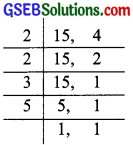LCM of 15 and 4 = 2 x 2 x 3 x 5 = 60
Since, the product of 15 and 4 = 60,
LCM of 15 and 4 = product of 15 and 4
We observe that:
(i) 36 = 3 x 12
(ii) 60 = 3 x 20
(iii) 30 = 3 x 10
i.e. LCM of any pair given here is a multiple of 3 Yes, in each case, we have:
LCM = product of the two numbersQuestion 11.
Find the LCM of the following numbers in which one number is the factor of the other
(a) 5, 20
(b) 6, 18
(c) 12, 48
(d) 9, 45
What do you observe in the results obtained?
Solution:
(a) 5 and 20
we haveLCM of 5 and 20 = 2 x 2 x 5 = 20

(b) 6 and 18
We haveLCM of 6 and 18 = 2 x 3 x 3 = 18

(c) 12 and 48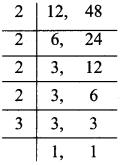LCM of 12 and 48 = 2 x 2 x 2 x 2 x 3 = 48

(d) 9 and 45
We have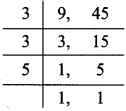LCM of 9 and 45 – 3 x 3 x 5 = 45
We observe that the LCM of the two numbers
[one is the factor of the other] is the greater number.